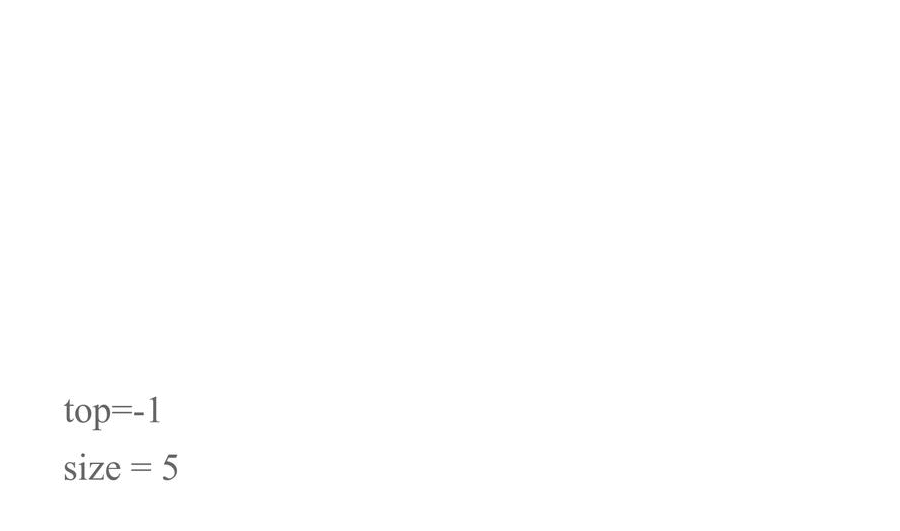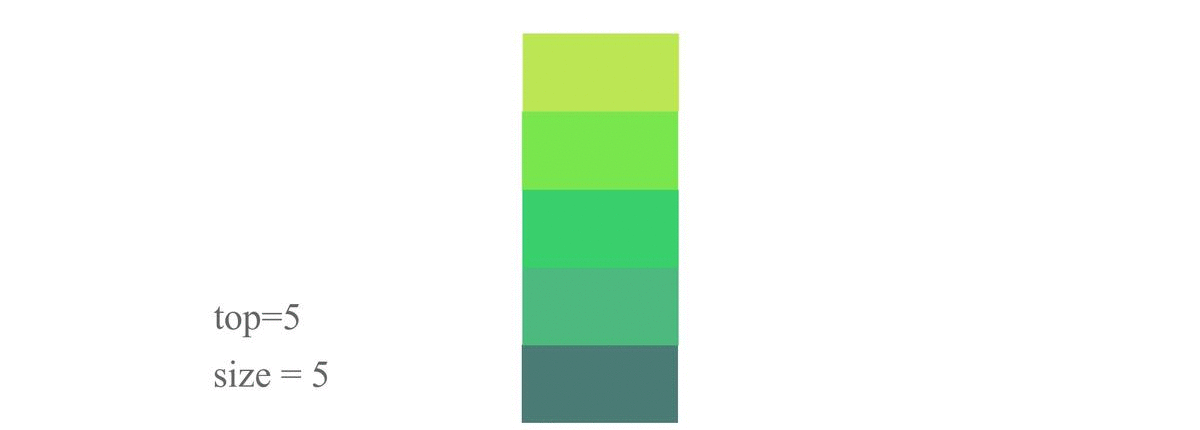# StacksCreated July 2, 2021

A stack is a linear data structure in which operations can be done in a specific order. Stacks follow the LIFO (Last In First Out) Principle.

I'm sure you might've heard of a stack of different things like a stack of papers or a stack of books. The stack data structure is exactly the same thing.The operations on a stack like - insertion or deletion can be done from only one end.

Like you might've noticed how we remove a book from a stack of number of books. If the required book present at the top then you just take that book out of the stack. This very operation in the stack data structure is called Pop operation.

Now if you would like to take a book out of that stack, which is present somewhere in the middle of that stack then you'll have to "Pop" all the books on top of it first until you reach the book you want. That is exactly how we search for items in stack data structure.

But we haven't yet talked about the creation of stack! So let's talk about that.

If we continue with my book stack analogy, creating a stack is nothing but putting one book on top of another book. It's as simple as that! And the operation of creation of stack involves the same procedure, it is known as Pushing. As in, if we want to insert an item after some other item in a stack, we say that we're "pushing" that element into the stack. I hope this gif makes the concept clearer -## Basic definition of Stack

Some of the main components of a stack are it's size, and the index of it's topmost element. We'll get to know what the importance of both in sometime. But let's first see how we define it in C.

``````typedef struct stack{
int arr[MAX];
int top;
int size;
}STACK;
``````

Here, MAX is a macro and I've set its value to 10. Stacks can be implemented using arrays as well as linked lists. We're gonna be doing array representation of linked lists in this article.

So, as we can see, arr[MAX] is just an array which is going to hold the items we store in the stack. Top will store the index value of the topmost element of the stack and size is equal to size of stack that the user inputs(we'll see that later in the code).

## Push

Push is the operation in stack data structure which inserts an element at the back of the stack.

So, for this operation, we just increase the value of top by one and insert the data at the top value index in the array of stack. The steps are:

1. Check if the stack is full or not.
2. If the stack is full - print "Overflow!". Otherwise, do the following:
1. Increase the value of top by one(top++)
2. Insert the value at the top index in the stack array(stack->arr[stack->top] == new item) The C code for the same is :
``````void push(STACK *s, int val){
if(s->top == s->size){
printf("STACK OVERFLOW!!\n");
}
else{
s->top++;
s->arr[s->top] = val;
}
}
``````

An illustration of the above code:## Pop

Pop is the process of deleting elements from a stack. Deletion in a stack can only be done from its rear end or we can say that only the topmost element can be deleted. For this operation we just decrease the value of top by one (top--). Steps followed:

1. Check if top is zero or not.
2. If top is zero then print "UNDERFLOW!". Otherwise, do the following:
1. Decrease the value of top by 1. A C code for the same looks something like this:
``````void pop(STACK *s){
if(s->top == 0){
printf("STACK UNDERFLOW!!\n");
}
else{
printf("Element Popped: %d\n", s->arr[s->top]);
s->top--;
}
}
``````If you observe carefully, in the Push operation, the last element to be pushed into the stack was the topmost element. In Pop operation, the first to be deleted was also the topmost element. This implies that the element that is pushed the last is deleted the first. That is called LIFO principle. The last element to enter is the first to exit.

## Peek

Peek is just to know which value is at the top index of the stack. All you have to do is print the topmost element. Steps:

1. Check if top is zero or not.
2. If not then print the topmost element. Otherwise, just print "Empty!". It can be done like this:
``````void peek(STACK *s){
if(s->top != 0){
printf("Topmost element is: %d\n", s->arr[s->top]);
}else{
printf("Empty!\n");
}
}
``````

So, now that we've discussed some basic stack functions let's take a look at the whole programme once.

``````#include <stdio.h>
#include <stdlib.h>
#define MAX 10
typedef struct stack{
int arr[MAX];
int top;
int size;
}STACK;

void push(STACK *s, int val){
if(s->top == s->size){
printf("STACK OVERFLOW!!\n");
}
else{
s->top++;
s->arr[s->top] = val;
}
}
void pop(STACK *s){
if(s->top == 0){
printf("STACK UNDERFLOW!!\n");
}
else{
printf("Element Popped: %d\n", s->arr[s->top]);
s->top--;
}
}
void peek(STACK *s){
if(s->top != 0){
printf("Topmost element is: %d\n", s->arr[s->top]);
}else{
printf("Empty!\n");
}
}

int main(){
int n,ch;
printf("enter the size of the stack: ");
scanf(" %d", &n);

STACK *s = (STACK*)malloc(sizeof(STACK));
s->top == -1;
s->size = n;

printf("\tSTACK IMPLEMENTATION!\n");
do{
printf("\tEnter: \n\t1.Push\n\t2.Pop\n\t3.Peek\n\t4.Exit\n");
printf("Enter Choice: ");
scanf("%d", &ch);
switch(ch){
case 1:
{
//push
printf("Enter item to push: ");
int val;
scanf(" %d", &val);
push(s,val);
break;
}
case 2:
{
//pop
pop(s);
break;
}
case 3:
{
//peek
peek(s);
break;
}
case 4:
{
//exit
printf("Exiting...\n");
break;
}
default:
{
printf("Enter Valid Choice!\n");
}
}

}while(ch!=4);

}
``````

Here, I've used the the do-while loop and switch case statements to call the functions as per the user's requirement.

## ...And that's it!

Hope you liked this article! You can find my other articles here.

Happy learning!